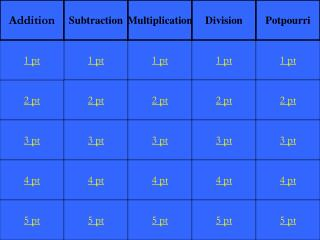DownloadDownload Presentation2 pt

# 2 pt

Download Presentation## 2 pt

- - - - - - - - - - - - - - - - - - - - - - - - - - - E N D - - - - - - - - - - - - - - - - - - - - - - - - - - -
##### Presentation Transcript

1. Addition Subtraction Multiplication Division Potpourri 1 pt 1 pt 1 pt 1 pt 1 pt 2 pt 2 pt 2 pt 2 pt 2 pt 3 pt 3 pt 3 pt 3 pt 3 pt 4 pt 4 pt 4 pt 4 pt 4 pt 5 pt 5 pt 5 pt 5 pt 5 pt

2. 568 + 74

3. What is 642

4. 2485 + 3874

5. What is 6359

6. 56.34 + 52.91

7. What is 109.25

8. 276 + 57 + 139

9. What is 472

10. What is

11. 471 - 59

12. What is 412

13. 1264 - 846

14. What is 418

15. 42.67 - 15.91

16. What is 26.76

17. 182.64 - 29.13

18. What is 153.51

19. What is

20. 15 x 4

21. What is 60

22. 45 x 3

23. What is 135

24. 36 x 8

25. What is 288

26. 18.7 x 6

27. What is 112.2

28. The number N stands for in the equation: N x 35 = 175

29. What is 60

30. What is 55

31. What is 8.6

32. What is 0.86

33. What is 30

34. All the factors of 6

35. What are 1, 2, 3, 6

36. The number of pints in a quart

37. The perimeter of a 2 x 4 rectangle

38. What is 12

39. The next 2 numbers in the pattern 3, 6, 12, ___, ___,

40. What are 24, 48

41. (14 x 3) + (75 - 50)